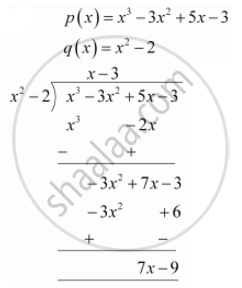# Divide the polynomial p(x) by the polynomial g(x) and find the quotient and remainder in each of the following p(x) = x^3 – 3x^2 + 5x – 3, g(x) = x2 – 2 - Mathematics

Divide the polynomial p(x) by the polynomial g(x) and find the quotient and remainder in each of the following

p(x) = x3 – 3x2 + 5x – 3, g(x) = x2 – 2

#### SolutionQuotient = x - 3

Remainder = 7x - 9

Concept: Division Algorithm for Polynomials
Is there an error in this question or solution?
Chapter 2: Polynomials - Exercise 2.3 [Page 36]

#### APPEARS IN

NCERT Class 10 Maths
Chapter 2 Polynomials
Exercise 2.3 | Q 1.1 | Page 36

Share